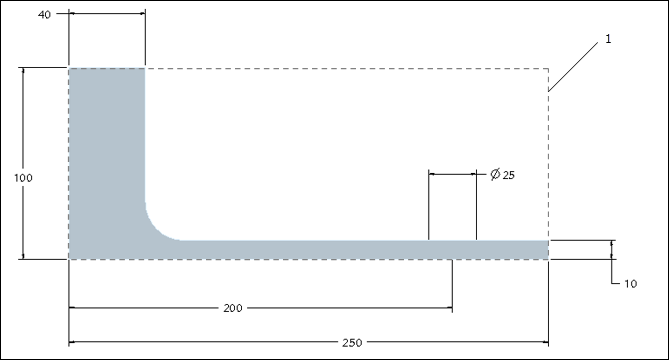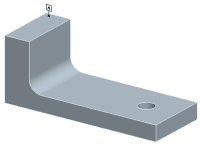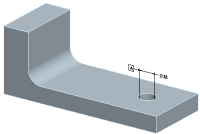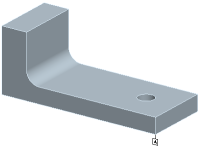The Primary Datum Feature May Be Too Small for the Design Model
The primary datum feature should generally be fairly large with respect to the size of the part or assembly model. When referenced in the predominant datum reference frame, the primary datum feature constrains the location and orientation of the part. If the datum feature is not sufficiently large, the constraint on the orientation of the part may not be sufficiently stable or repeatable.
For each orientation DOF of the part that is constrained by the primary datum feature, GD&T Advisor uses the following procedure to determine if the primary datum feature of the predominate datum reference frame is suitable for the part:
1. Find a plane whose normal is parallel to the axis of rotation of the constrained DOF.
2. Find: Max_Proj_Part_Dim (the maximum dimension of the projection of the part bounding box onto that plane).
3. Find: Char_Len (the applicable dimension of the datum feature that is related to the constrained DOF).
4. Calculate: DimRatio = Max_Proj_Part_Dim/Char_Len.
5. Show message if (DimRatio > PRT_PrimDFSize), where PRT_PrimDFSize is defined in the application options.
If you see this advisor message, you should consider setting a different primary datum feature.
Example
Consider the example shown in the picture below:1. Bounding box (250x100x90)
The table below shows a few possible candidates for the primary datum feature for this part and the status for this test, assuming PRT_PrimDFSize is set to 5.0 in the application options.
Example
Test Evaluation
Test StatusChar_Len for Primary DF:
Char_Len(RX)=90
Char_Len(RZ)=40
Ratios:
DimRatio(RX)=90/90=1.0
DimRatio(RZ)=250/40=6.25
Fail because:
DimRatio(RZ)>PRT_PrimDFSizeChar_Len for Primary DF:
Char_Len(RX)=25
Char_Len(RZ)=25
Ratios:
DimRatio(RX)=90/25=3.2
DimRatio(RZ)=250/25=10.0
Fail because:
DimRatio(RZ)>PRT_PrimDFSizeChar_Len for Primary DF:
Char_Len(RX)=90
Char_Len(RZ)=250
Ratios:
DimRatio(RX)=90/90=1.0
DimRatio(RZ)=250/250=1.0
Pass because:
DimRatio(RX)<PRT_PrimDFSize AND
DimRatio(RZ)<PRT_PrimDFSize
In this case, the bottom planar surface of the part would probably be the best choice as the primary datum feature.